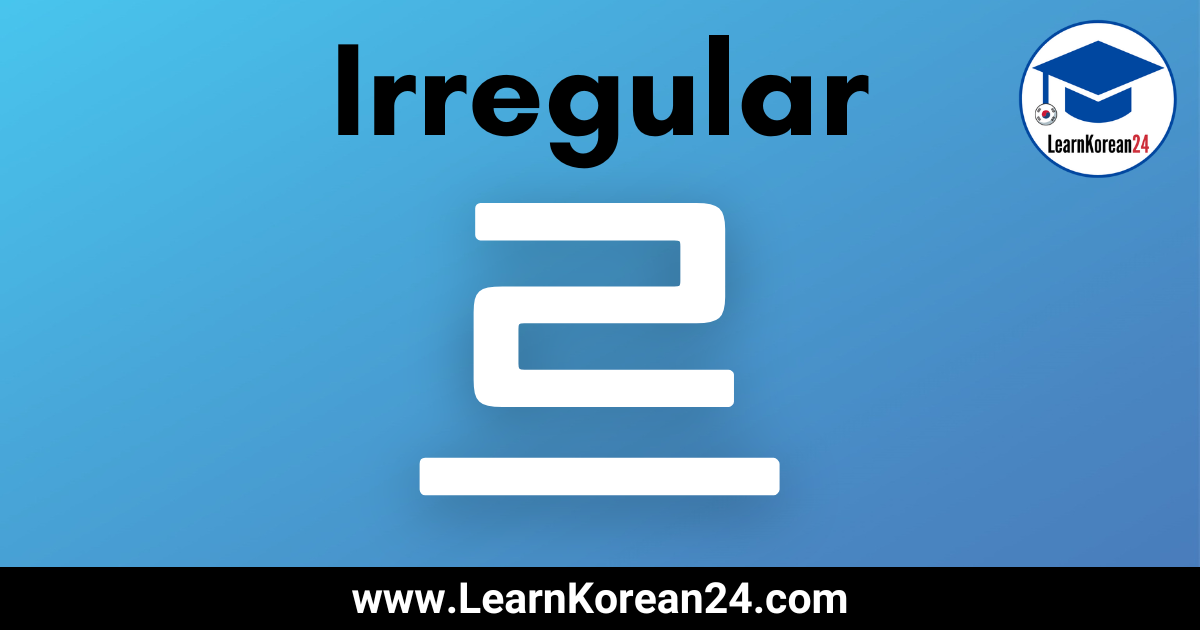# Irregular Korean Verbs With ‘르’ (르 불규칙)In this lesson, you will learn how to conjugate irregular verbs with 르 (르 불규칙). In a previous lesson, you learned about ㅡ irregular verbs. Although 르 irregular verbs also end in ㅡ, they are different and follow different conjugation rules.

Below you will find a list of 르 irregular verbs and will learn how to conjugate irregular verbs with 르.

## 르 Irregular (르 불규칙)

Verbs and adjectives that end in 르 are irregular. This means that when they are conjugated with various endings, they do not follow the normal conjugation rules. For example, the adjective 빠다 (to be fast) ends in and so it is irregular. When conjugating 빠르다 in the present tense it becomes 빨라요 and not 빨르어요.

Here is a list of 르 irregular verbs in Korean (르 불규칙):

• 빠르다 = to be fast
• 부르다 = to call/sing
• 고르다 = to choose
• 기르다 = to raise/grow
• 나르다 = to carry
• 누르다 = to press
• 다르다 = to be different
• 바르다 = to apply
• 오르다 = to go up/climb
• 이르다 = to be early
• 자르다 = to cut
• 흐르다 = to flow
• 서두르다 = to hurry
• 서투르다 = to be clumsy
• 배부르다 = to be full
• 가르다 = to divide
• 구르다 = to roll
• 모르다 = to not know

## How To Conjugate ‘르’ Irregular Verbs (르 불규칙)

If you have read the previous lesson about ㅡ irregular verbs, then learning how to conjugate 르 irregular verbs is not very difficult. Here are the key things to remember when conjugating irregular verbs that end in 르:

1. Irregular verbs and adjectives ending in 르 only behave irregularly when an ending starting with 아 or 어 is attached.

For example, endings such as 아요/어요 (present tense), 았어요/었어요 (past tense), etc, start with 아 or 어. So, when these endings are attached to 르 irregular verbs and adjectives it makes them behave irregularly. That is to say, they are conjugated differently than regular verbs and adjectives.

When 르 irregular verbs and adjectives are conjugated with endings which do not start with 아 or 어, they do not behave irregularly and are just conjugated the same as normal verbs and adjectives.

2. When conjugating irregular 르 verbs and adjectives with endings starting with 아 or 어, ㅡ is always dropped and an additional ㄹ is added.

Take the verb 모르다, for example. When conjugated in the present tense, 모르다 becomes 몰라요. This is because ㅡ is dropped and an additional ㄹ is added before the ending 아요.

3. The vowel that comes before 르 determines whether 아 or 어 is used in the ending.

For example, when you want to conjugate an irregular 르 verb with the ending 아서/어서 (so), you must choose to add 아서 or 어서. The one you should choose is determined by the vowel which precedes 르 in the verb/adjective stem.

If the vowel before 르 is ㅏ or ㅗ, then the ending starting with 아 should be used.

For example, the vowel before 르 in 빠르다 (to be fast) is ㅏ. So, the ending starting with 아 should be used.

빠르다 = to be fast
빨라요 = fast (present tense)
빨랐어요 = was fast (past tense)
빨라서… = fast, so…

If the vowel before 르 is NOT ㅏ or ㅗ, then the ending starting with 어 should be used.

For example, the vowel before 르 in the verb 부르다 (to sing/call) is ㅜ. As this is not ㅏ or ㅗ, we should choose the ending starting with 어.

부르다 = to sing/call
불러요 = sing/call (present tense)
불렀어요 = sang/called (past tense)
불러서… = sing, so…

4. The last thing to know about 르 irregular verbs is that there are a few exceptions.

There are a few exceptions to the above conjugation rules. The following verbs all look like irregular 르 verbs, but they are actually irregular ㅡ verbs. And so they should be conjugated the same way as irregular ㅡ verbs (i.e. no additional ㄹ is added).

따르다 = to follow
치르다 = to pay
들르다 = to drop in

## Irregular 르 Examples

Here are some examples of irregular verbs and adjectives that end in 르 conjugated with some common endings.

## Irregular 르 Example Sentences

Here are some example sentences which include irregular 르 verbs.

• BTS 를 정말 몰라요? (모르다) = You really don’t know BTS?
• 제 친구는 화초를 길러요. (기르다) = My friend grows plants.
• 머리를 얼마나 자주 잘라요? (자르다) = How often do you get your haircut?
• 택시가 버스보다 더 빨라요. (빠르다) = Taxis are faster than buses.
• 목이 아파서 노래를 못 불러요 (부르다) = I have a sore throat so I can’t sing.

## More Korean Irregular Verb Lessons

There are seven types of irregular verbs in Korean. For more lessons on Korean Irregular verbs, click on the links below.